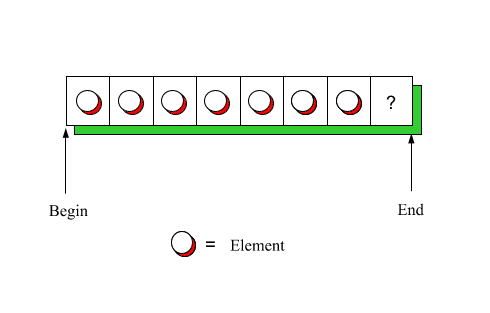DOC HOME SITE MAP MAN PAGES GNU INFO SEARCH PRINT BOOK
No More Array Errors (Part II) - Array_alg(C++)

# The Array_set representation

Using an array (or Block) to represent Array_set means that its contents must satisfy the simple invariant ``no repetitions.'' To minimize the cost of maintaining the invariant, we will keep the array sorted at all times. The representation invariant will therefore have two terms:

sortedness:
i <= j implies x[i] <= x[j]

uniqueness:
i != j implies x[i] != x[j]

The only way to guarantee invariance is to create an abstract data type. An abstract data type protects data from access or modification except by its own operations, which cooperate in maintaining the invariant.

Since Array_sets are parameterized by their element type, we need to define the following template class definition:

```   Array_set.h

template <class T> class Array_set{
...
};
template <class T> class Array_setiter{
...
};

template <class T>        Array_set<T>::Array_set(){...}
...
ostream& operator<<(ostream os,
const Array_set<T>& s){..}

```

To simplify memory management, we will use a Block<T> instead of an array as the underlying representation of Array_set<T>. Furthermore, we will use Array Algorithms defined in Array_alg.h:

```     Array_set.h

#include <Block.h>
#include <Array_alg.h>

template <class T> class Array_set{
private:
Block<T> b;
...
```

We are immediately faced with an efficiency issue: should b have exactly enough space for the elements of the Array_set, and no more? This would imply that an empty Array_set would be created with a Block of size zero, and the size of the Array_set would at all times be given by the size of b:

```       template <class T> class Array_set{
private:
Block<T> b;
public:
Array_set():b(0){ }
unsigned size()const{
return b.size();
}
...
};
```

If we adopt this representation, however, we must reallocate b each time an element is added or removed from the Array_set. For example:

```        const T* insert(const T& t,int count=1){
...
if(  t is not already present  ){
b.size(b.size()+1);
insert t         }
}
```
```        unsigned remove(const T& t,int count=1){
...
if(  t is present  ){
b.size(b.size()-1);
remove t         }
}
```

The count parameter is present so that Sets and Bags (which allow multiple occurrences) can share a common interface. For Sets, specifying a value of count greater than one should have the same effect as specifying the value one. The default value allows clients to ignore the parameter.

Reallocation, however, is an expensive operation: according to Block(C++), size() allocates a new region of memory having exactly the number of cells requested, copies the values from the old region to the new region, and deletes the old region. To avoid the expense of reallocation, the Array_set constructors will allocate b with excess capacity:

```       template <class T> class Array_set{
private:
Block<T> b;
public:
Array_set():b(10){ }
unsigned size()const{
return  ??         }
...
};
```

Now, however, the size of the Array_set is no longer given by the size of b. We must therefore add a data member that keeps track of the number of elements in the Array_set (n satisfies the invariant 0 <= n<b.size() ):

```       template <class T> class Array_set{
private:
Block<T> b;
unsigned n;
public:
Array_set():b(10),n(0){ }
unsigned size()const{
return n;
}
};
```

Before proceeding, let's formalize the representation invariant. This is helpful, not only in understanding the problem and helping explain it to others, but also in debugging, since runtime invariant checks usually catch programming errors at their source. We will write a private function called check() to check the invariant. (We use the assert(3) mechanism so that checking can be turned on or off by a compile-time switch. When turned off, the inline function check() will have an empty body and call to it will (hopefully) be optimized away by the compiler.)

```       template <class T> class Array_set{
private:
Block<T> b;
unsigned n;
void check(){
check that n <= b.size()
check that i<j implies b[i] <= b[j]
check that i != j implies b[i] != x[j]         }
...
```

Checking the first term of the invariant is straightforward.

```        assert(n<=b.size());
```

How do we check the two remaining terms? The second term could be checked by looping over the n elements of b and comparing each element to the preceding element, if any. Instead, we will use an Array Algorithm.

The Array Algorithm generate(), which is described in generate(C++), calls a programmer-defined function for each element of an array, from first to last. We will use generate() to apply a function called compare() to each element of b.

```        static void compare(ptrdiff_t n,T* p){
assert(n==0 || *p >= *(p-1));
}
```
```       template <class T> class Array_set{
private:
Block<T> b;
unsigned n
void check(){
assert(n<=b.size());
generate(compare,&b,&b[n]);
check that i != j implies b[i] != x[j]        }
```

Now that the second term has been checked, we know that the elements between &b and &b[n-1] are in sorted order.

We can then use an algorithm that assumes sortedness to check for duplicates. We will use the Array Algorithm unique(), one of four algorithms described in unique(C++), to do this. unique() is designed to remove duplicate elements from a sorted array in a single pass:

```            void check(){
assert(n<=b.size());
generate(compare,&b,&b[n]);
assert(unique(&b,&b[n])==&b[n]);
}
```

generate() and unique() illustrate a convention followed by all the Array Algorithms: an array is identified by a pair of pointers: the first pointer points to the first element, and the second pointer points just beyond the last element:Next topic: Array_set insertion
Previous topic: An array-based implementation of Set(C++)